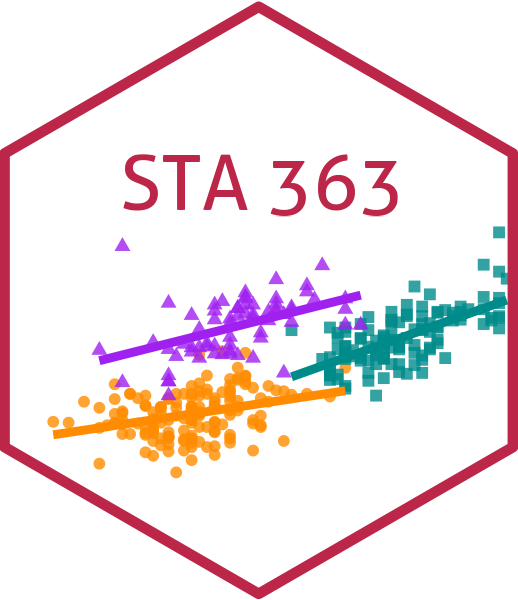# Lasso and Elastic Net

## Ridge Review

What are we minimizing with Ridge Regression?

• $RSS + \lambda\sum_{j=1}^p\beta_j^2$

## Ridge Regression

What is the resulting estimate for $\hat\beta_{ridge}$?

• $\hat\beta_{ridge} = (\mathbf{X}^{T}\mathbf{X}+\lambda\mathbf{I})^{-1}\mathbf{X}^T\mathbf{y}$

## Ridge Regression

Why is this useful?

## Ridge Review

How is $\lambda$ determined?

$RSS + \lambda\sum_{j=1}^p\beta_j^2$

## Ridge Regression

### Pros

• Can be used when $p > n$
• Can be used to help with multicollinearity
• Will decrease variance (as $\lambda \rightarrow \infty$ )

### Cons

• Will have increased bias (compared to least squares)
• Does not really help with variable selection (all variables are included in some regard, even if their $\beta$ coefficients are really small)

## Lasso!

• The lasso is similar to ridge, but it actually drives some $\beta$ coefficients to 0! (So it helps with variable selection)
• $RSS + \lambda\sum_{j=1}^p|\beta_j|$
• We say lasso uses an $\ell_1$ penalty, ridge uses an $\ell_2$ penalty
• $||\beta||_1=\sum|\beta_j|$
• $||\beta||_2=\sum\beta_j^2$

## Lasso

• Like Ridge regression, lasso shrinks the coefficients towards 0
• In lasso, the $\ell_1$ penalty forces some of the coefficient estimates to be exactly zero when the tuning parameter $\lambda$ is sufficiently large
• Therefore, lasso can be used for variable selection
• The lasso can help create smaller, simplier models
• Choosing $\lambda$ again is done via cross-validation

## Lasso

### Pros

• Can be used when $p > n$
• Can be used to help with multicollinearity
• Will decrease variance (as $\lambda \rightarrow \infty$ )
• Can be used for variable selection, since it will make some $\beta$ coefficients exactly 0

### Cons

• Will have increased bias (compared to least squares)
• If $p>n$ the lasso can select at most $n$ variables

## Ridge versus lasso

• Neither Ridge nor lasso will universally dominate
• Cross-validation can also be used to determine which method (Ridge or lasso) should be used
• Cross-validation is also used to select $\lambda$ in either method. You choose the $\lambda$ value for which the cross-validation model is the smallest

## What if we want to do both?

• Elastic net!

• $RSS + \lambda_1\sum_{j=1}^p\beta^2_j+\lambda_2\sum_{j=1}^p|\beta_j|$

What is the $\ell_1$ part of the penalty?

What is the $\ell_2$ part of the penalty

## Elastic net

$RSS + \lambda_1\sum_{j=1}^p\beta^2_j+\lambda_2\sum_{j=1}^p|\beta_j|$

When will this be equivalent to Ridge Regression?

## Elastic net

$RSS + \lambda_1\sum_{j=1}^p\beta^2_j+\lambda_2\sum_{j=1}^p|\beta_j|$

When will this be equivalent to Lasso?

## Elastic Net

$RSS + \lambda_1\sum_{j=1}^p\beta^2_j+\lambda_2\sum_{j=1}^p|\beta_j|$

• The $\ell_1$ part of the penalty will generate a sparse model (shrink some $\beta$ coefficients to exactly 0)
• The $\ell_2$ part of the penalty removes the limitation on the number of variables selected (can be $>n$ now)

How do you think $\lambda_1$ and $\lambda_2$ are chosen?